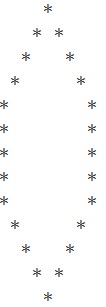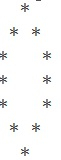# C Program for Hexagonal Pattern

We are given with an integer ‘n’ and the task is to generate the hexagonal pattern and display the final output.

## Example

Input-: n=5
Output-:Input-: n = 4
Output-:Approach we are using in the given program is as follows

• Input the number ‘n’ from user
• Divide the entire pattern into three parts i.e. upper part, middle part and lower part Start loop i for printing the upper part of the pattern from i to 0 and i to be less than n and keep incrementing the value of i Start loop m for printing the middle part of the pattern from m to 0 and m to be less than n-2 and keep incrementing the value of m Start loop h for printing the lower part of the pattern from h to res and h to be greater than 0 and keep decrementing the value of h Keep printing the * with spaces.

## ALGORITHM

START
Step 1-> declare function to print hexagonal pattern
void pattern(int n)
Declare and set int len = 2 * n - 1
Loop For int i = 0 and i < n and i++
declare and set int temp = i + n
Loop For int k = 0 and k < temp and k++
IF ((k == n + i - 1) || (k == n - i - 1))
print *
End
Else
print space
End
Print
End
Loop For int m = 0 and m < n - 2 and m++
Loop For int j = 0 and j < len and j++
if (j == 0 || j == len - 1)
Print *
End
Else
print space
End
End
Print
End
declare and set int res = n - 1
Loop For int h = res and h >= 0 and h--
declare and set int temp2 = h + n
Loop For int k = 0 and k < temp2 and k++
if ((k == n + h - 1) || (k == n - h - 1))
print *
End
Else
print space
End
End
Print
End
End
Step 2-> In main()
Declare variable int n = 5
call pattern(n)
STOP

## Example

#include <stdio.h>
//program to print hexagon pattern
void pattern(int n) {
int len = 2 * n - 1;
//for loop for upper part of a pattern
for (int i = 0; i < n; i++) {
int temp = i + n;
for (int k = 0; k < temp; k++) {
if ((k == n + i - 1) || (k == n - i - 1))
printf("*");
else
printf(" ");
}
printf("");
}
//for loop for mid part of a pattern
for (int m = 0; m < n - 2; m++) {
for (int j = 0; j < len; j++) {
if (j == 0 || j == len - 1)
printf("*");
else
printf(" ");
}
printf("");
}
//for loop for lower part of a pattern
int res = n - 1;
for (int h = res; h >= 0; h--) {
int temp2 = h + n;
for (int k = 0; k < temp2; k++) {
if ((k == n + h - 1) || (k == n - h - 1))
printf("*");
else
printf(" ");
}
printf("");
}
}
int main() {
int n = 5;
pattern(n);
return 0;
}

## Output